Question

# Conduct the following test at the alphaαequals=0.10 level of significance by determining ​(a) the null and...

Conduct the following test at the alphaαequals=0.10 level of significance by determining ​(a) the null and alternative​ hypotheses, ​(b) the test​ statistic, and​ (c) the​ P-value. Assume that the samples were obtained independently using simple random sampling. Test whether p1≠p2. Sample data are x1=28​, n1=254​, x2=38​, and n2=301.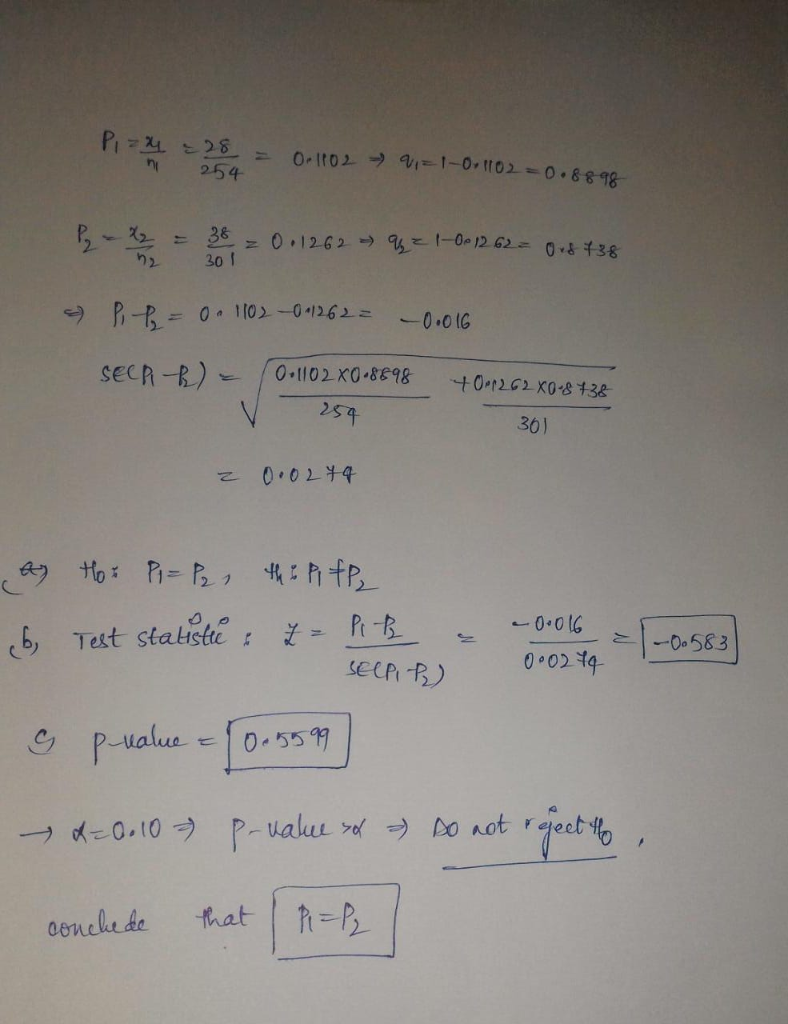#### Earn Coins

Coins can be redeemed for fabulous gifts.

Similar Homework Help Questions
• ### Conduct a test at the alphaαequals=0.10 level of significance by determining ​(a) the null and alternative​...

Conduct a test at the alphaαequals=0.10 level of significance by determining ​(a) the null and alternative​ hypotheses, ​(b) the test​ statistic, and​ (c) the​ P-value. Assume the samples were obtained independently from a large population using simple random sampling. Test whether p1>p2. The sample data are x1=119​, n1=257​, x x2=137​, and n2=306. Determine the test statistic and the P-value

• ### Conduct a test at the a=0.01 level of significance by determining (a) the null and alternative...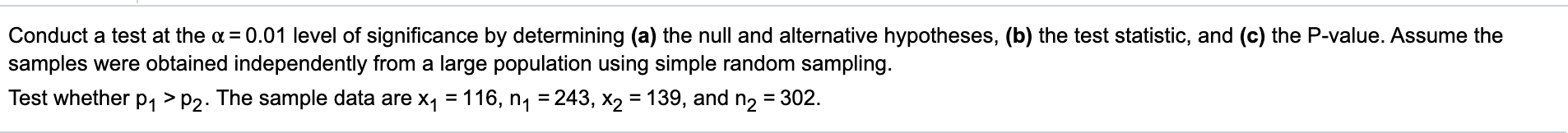Conduct a test at the a=0.01 level of significance by determining (a) the null and alternative hypotheses, (b) the test statistic, and (c) the P-value. Assume the samples were obtained independently from a large population using simple random sampling. Test whether P1 >P2. The sample data are x1 = 116, n1 = 243, X2 = 139, and n2 = 302.

• ### Conduct the following test at the a = 0.10 level of significance by determining (a) the...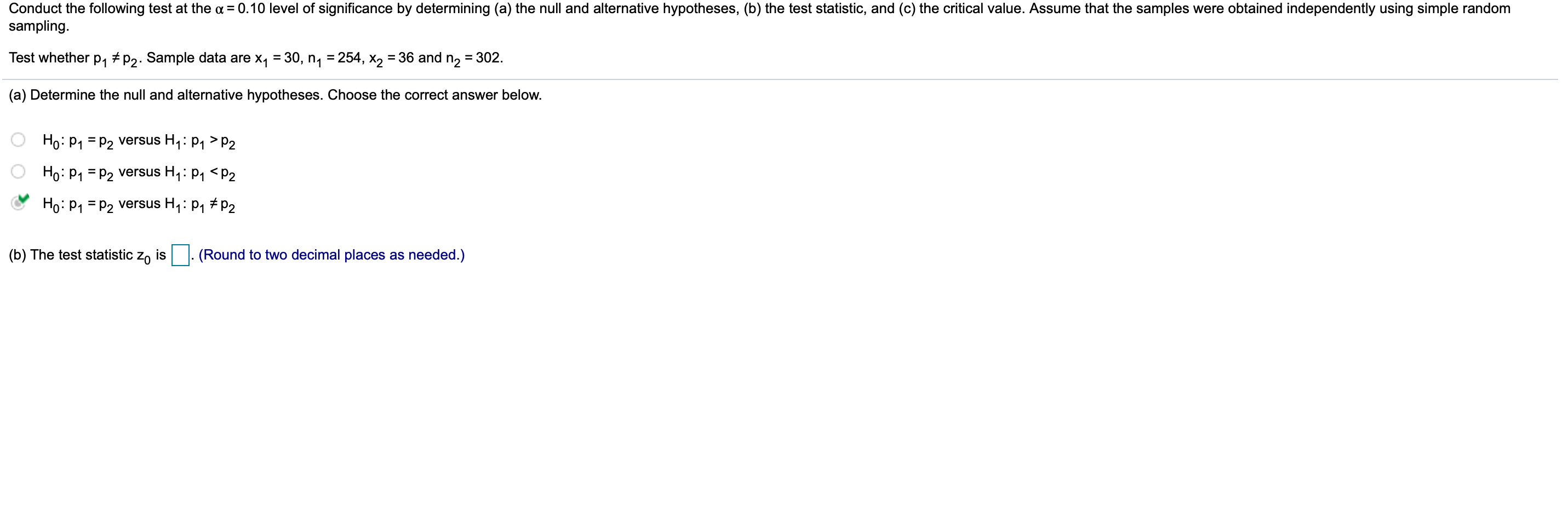Conduct the following test at the a = 0.10 level of significance by determining (a) the null and alternative hypotheses, (b) the test statistic, and (c) the critical value. Assume that the samples were obtained independently using simple random sampling. Test whether P1 7P2. Sample data are X7 = 30, ny = 254, X2 = 36 and n2 = 302. (a) Determine the null and alternative hypotheses. Choose the correct answer below. Ho: P1 = P2 versus Hy: P1 >P2...

• ### Round to six decimal places as needed. Conduct the following test at the a = 0.01...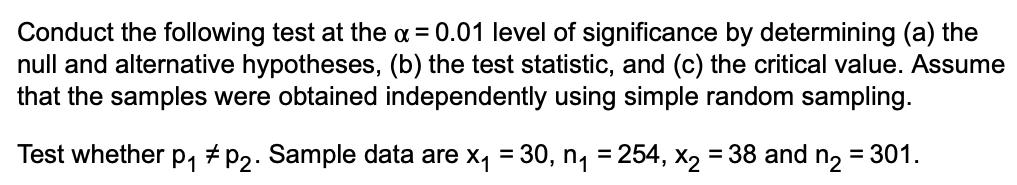Round to six decimal places as needed. Conduct the following test at the a = 0.01 level of significance by determining (a) the null and alternative hypotheses, (b) the test statistic, and (c) the critical value. Assume that the samples were obtained independently using simple random sampling. Test whether P1 * P2. Sample data are x1 = 30, ny = 254, X2 = 38 and n2 = 301.

• ### Conduct a test at the a = 0.10 level of significance by determining (a) the null...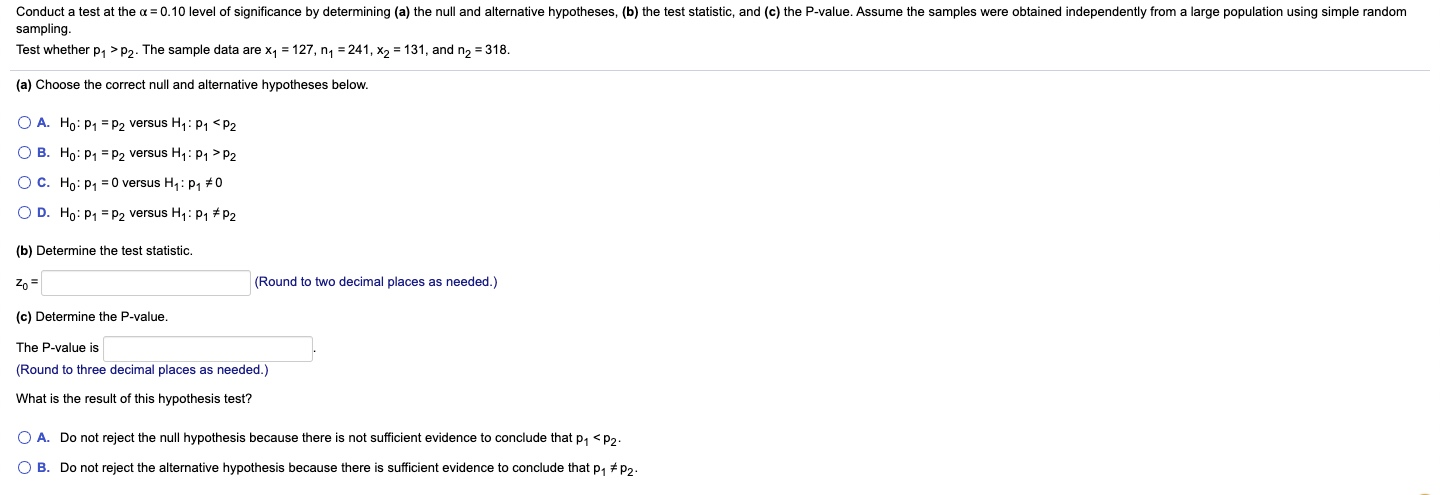Conduct a test at the a = 0.10 level of significance by determining (a) the null and alternative hypotheses, (b) the test statistic, and (c) the P-value. Assume the samples were obtained independently from a large population using simple random sampling. Test whether 21 > P2. The sample data are x1 = 127, ny = 241, X2 = 131, and n2 = 318. (a) Choose the correct null and alternative hypotheses below. O A. Ho: P1 = P2 versus Hy:...

• ### Conduct the following test at the a = 0.01 level of significance by determining (a) the...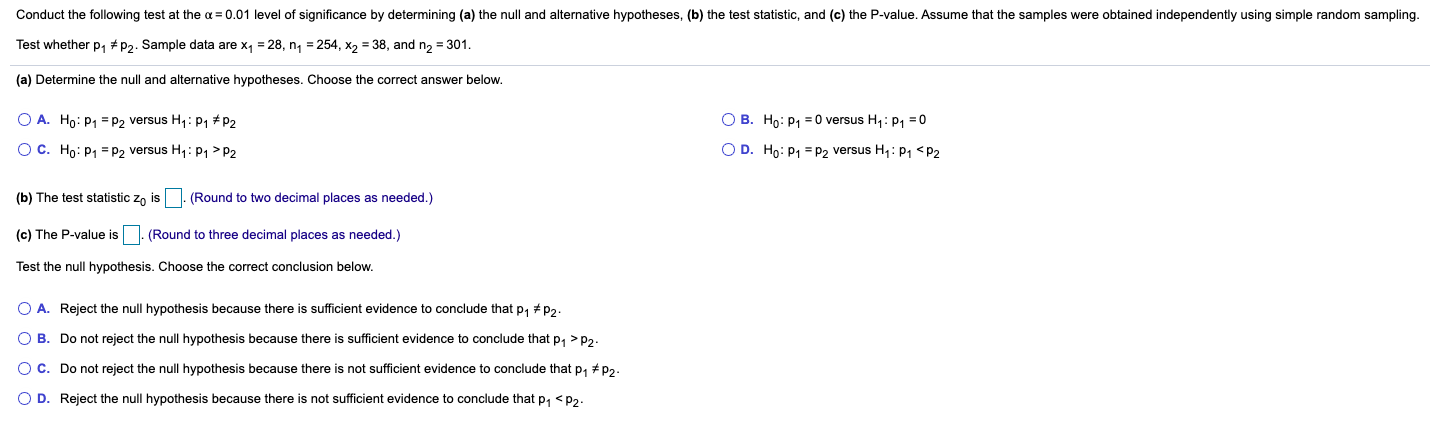Conduct the following test at the a = 0.01 level of significance by determining (a) the null and alternative hypotheses, (b) the test statistic, and (c) the P-value. Assume that the samples were obtained independently using simple random sampling. Test whether P1 * P2. Sample data are xy = 28, n = 254, X2 = 38, and n2 = 301. (a) Determine the null and alternative hypotheses. Choose the correct answer below. O A. Ho: P1 = P2 versus Hy:...

• ### Conduct a test at the alpha equals 0.05 level of significance by determining ​(a) the null...

Conduct a test at the alpha equals 0.05 level of significance by determining ​(a) the null and alternative​ hypotheses, ​(b) the test​ statistic, and​ (c) the​ P-value. Assume the samples were obtained independently from a large population using simple random sampling. Test whether p1 greater than p2. The sample data are x1 = 123​, n 1 = 242​, x2 = 137​, and n2 = 312. If there is anyway you can explain how to get the answer via Stat Crunch,...

• ### Conduct the following test at the a = 0.05 level of significance by determining (a) the...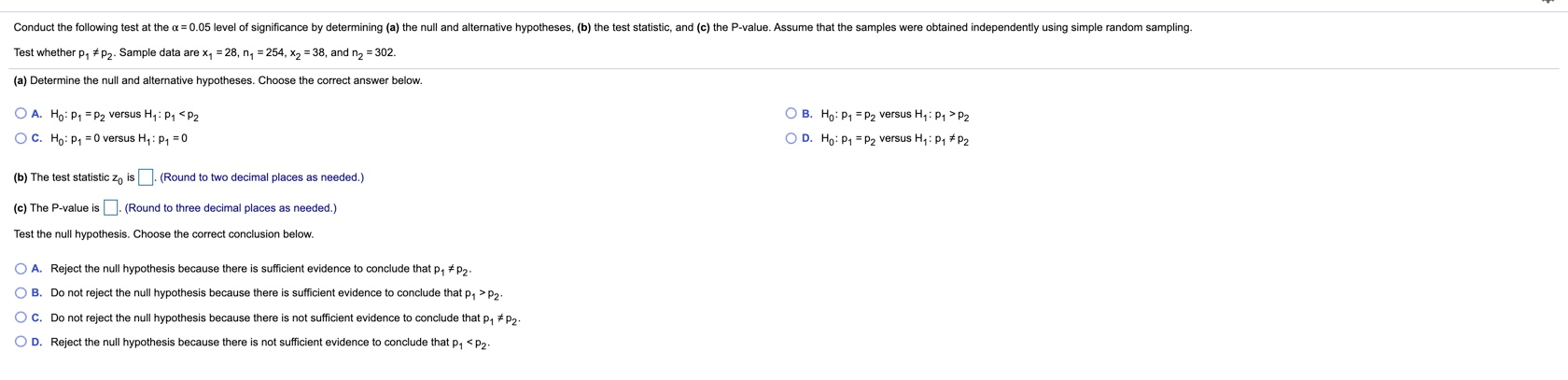Conduct the following test at the a = 0.05 level of significance by determining (a) the null and alternative hypotheses, (b) the test statistic, and (c) the P-value. Assume that the samples were obtained independently using simple random sampling. Test whether P1 * P2. Sample data are x, = 28, ny = 254, X2 = 38, and n2 = 302. (a) Determine the null and alternative hypotheses. Choose the correct answer below. OB. Ho: P = P2 versus HP1 P2...

• ### Conduct the following test at the a = 0.01 level of significance by determining (a) the...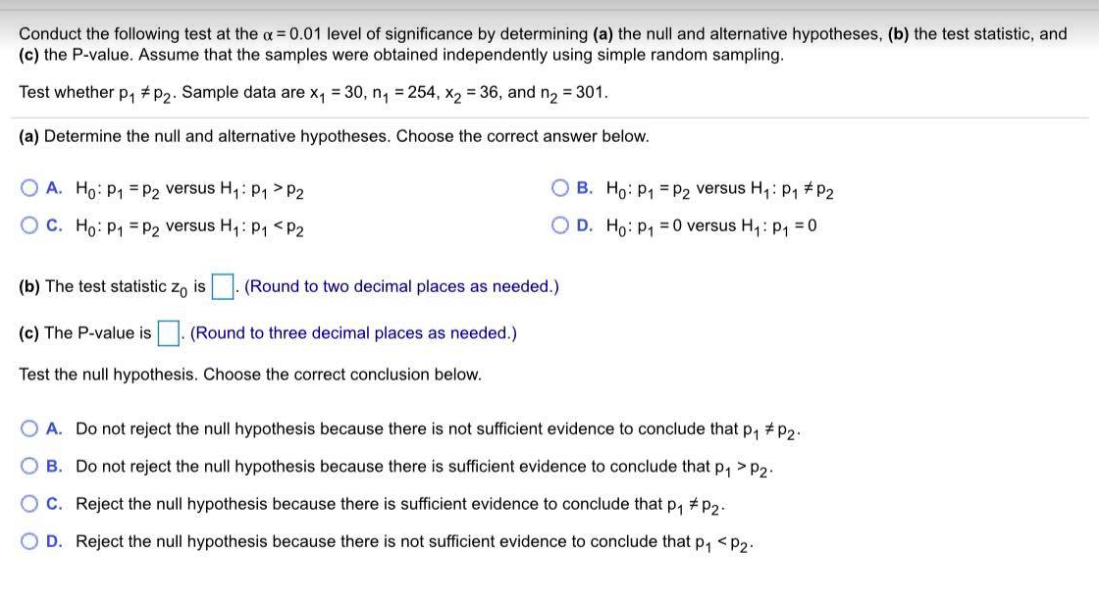Conduct the following test at the a = 0.01 level of significance by determining (a) the null and alternative hypotheses, (b) the test statistic, and (c) the P-value. Assume that the samples were obtained independently using simple random sampling. Test whether p1 #P2. Sample data are X7 = 30, n = 254, X2 = 36, and n2 = 301. (a) Determine the null and alternative hypotheses. Choose the correct answer below. O A. Ho: P1 = P2 versus H: P1>P2...

• ### Conduct a test at the alphaα=0.05 level of significance by determining ​(a) the null and alternative​...

Conduct a test at the alphaα=0.05 level of significance by determining ​(a) the null and alternative​ hypotheses, ​(b) the test​ statistic, and​ (c) the​ P-value. Assume the samples were obtained independently from a large population using simple random sampling. Test whether p 1 > p 2. The sample data are x1=120​, n1=246​, x2=132​, and n2=307. a) Determine the test statistic. z0= ​(Round to two decimal places as​ needed.) b) Determine the p-value (Round to three decimal places b) Determine the...